PSABM6NK - Student Facing Task---Acc7.3 Lesson 8 Reasoning about Solving Equations (Part 2) (6.EE.A.4, 7.EE.B.4.a)

Select all the expressions equivalent to 2(x + 3).

Select all that apply:
Part A)

Explain why either of the following equations could represent the hanger.or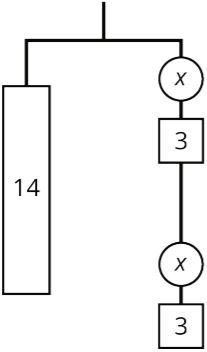Part B)

Find the weight of one circle.

x = ______

Type your answer below as a number (example: 5, 3.1, 4 1/2, or 3/2):
Part A)

Each piece is labeled with its weight. Which equation matches with this hanger?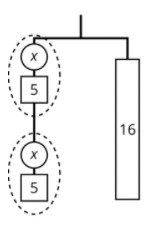Select one:
Part B)

Each piece is labeled with its weight. Which equation matches with this hanger?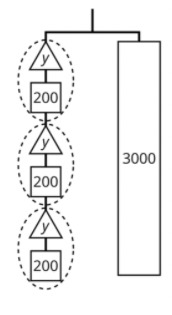Select one:
Part C)

Each piece is labeled with its weight. Which equation matches with this hanger?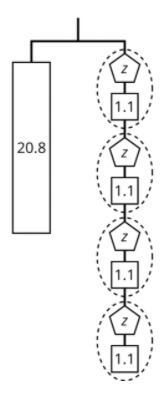Select one:
Part D)

Each piece is labeled with its weight. Which equation matches with this hanger?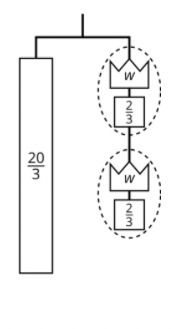Select one:
Part E)

Explain how to figure out the weight of a piece labeled with a letter by reasoning about the diagram.